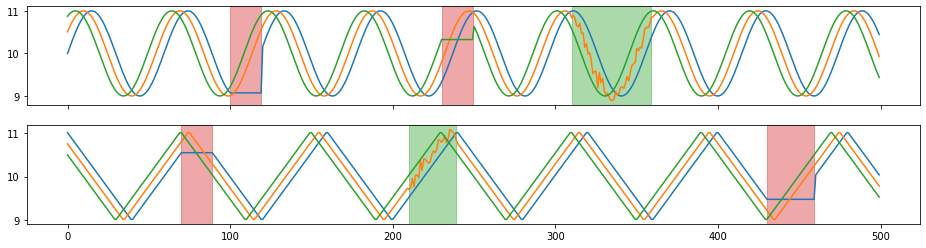# Examples of Augmenters¶

In this notebook, we demonstrate every augmenter by applying it to a batch of synthetic multivariate time series and to a 2-channel audio sequence.

:

import numpy as np

import librosa
from IPython.display import Audio

import tsaug
from tsaug.visualization import plot

:

X = np.load("./X.npy")

plot(X, Y);:

beethoven, samplerate = librosa.load("./beethoven.wav", mono=False)
beethoven = np.expand_dims(beethoven.swapaxes(0,1), 0) # reshape the numpy array to input of tsaug

plot(beethoven);

Audio(beethoven.reshape(-1, 2).T, rate=samplerate)

: